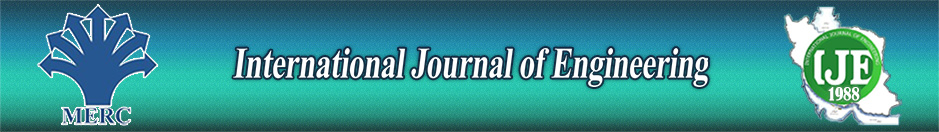Analytical Investigation of Forced Convection in Thermally Developed Region of a Channel Partially Filled with an Asymmetric Porous Material- LTNE Model

Author

Department of Renewable Energy, Material and Energy Research Center(MERC)

Abstract

In present work forced convection flow in a channel partly filled with a porous media under asymmetric heat flux boundary condition has been investigated. The porous material is distributed on the one wall. Darcy–Brinkman and LTNE model have been assumed in order to solve momentum and energy equations, respectively. Fully developed conditions are considered in order to solve velocity and the temperature fields. Due to different configuration of porous media new velocity temperature profiles are introduced. The Nusselt number (Nu) is deduced in terms of the porous insert thickness(S), thermal conductivity ratio (k) and Darcy number (Da). The results illustrate that the fluid temperature in the clear region declines when the porous thickness grows at constant k.  The temperature difference between the fluid phase and the solid phase is decrease, as Bi increases. There are two stages for variation of Nu number with S and the optimum Nusselt thicknesses of the porous medium Sopt,Nu is found for different value of k and Bi. Moreover, at the particular value of S Nu number is independent of Bi and k for each Da number.

Keywords